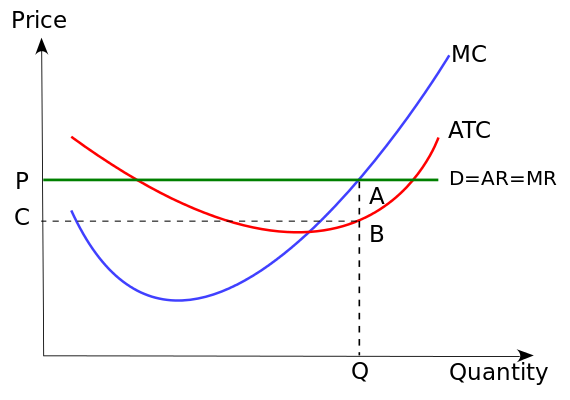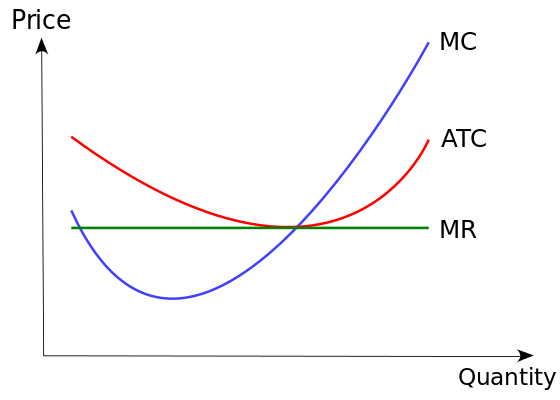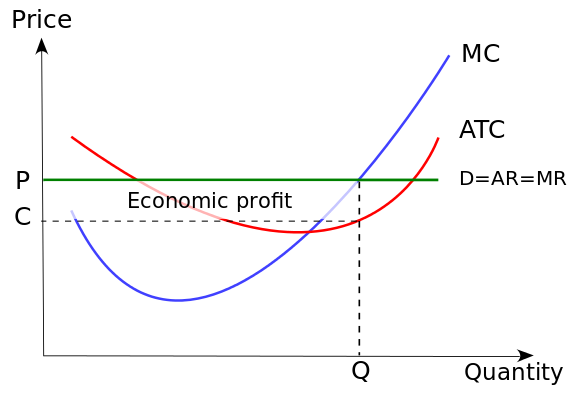# 10.2: Production Decisions in Perfect Competition

## Relationship Between Output and Revenue

Output is the amount of a good produced; revenue is the amount of income made from sales minus all business expenses.

learning objectives

• Describe the relationship between output and revenue

### Output

In economics, output is defined as the quantity of goods or services produced in a certain period of time by a firm, industry, or country. Output can be consumed or used for further production. Output is important on a business and national scale because it is output, not large sums of money, that makes a company or country wealthy.

There are many factors that influence the level of output including changes in labor, capital, and the efficiency of the factors of production. Anything that causes one of the factors to increase or decrease will change the output in the same manner.

### Revenue

Revenue, also known as turnover, is the income that a company receives from normal business activities, usually from the sale of goods and services. Revenue is the money that is made as a result of output, or amount of goods produced. Companies can also receive revenue from interest, royalties, and other fees.

Revenue can refer to general business income, but it can also refer to the amount of money made during a specific time period. When companies produce a certain quantity of a good (output), the revenue is the amount of income made from sales during a set time period.

Businesses analyze revenue in their financial statements. The performance of a company is determined by how its asset inflows (revenues) compare with its asset outflows (expenses). Revenue is an important financial indiator, though it is important to note that companies are profit maximizers, not revenue maximizers.

### Importance of Output and Revenue

In order for a company or firm to be successful, it must focus on both the output and revenue. The quantity of goods produced must meet public demand, but the company must also be able to sell those goods in order to generate revenue. The production of goods carries a cost, so companies want to find a level of output that maximizes profit, not revenue.Output and Revenue: Krispy Kreme’s output is donuts. It generates revenue by selling its output. It is however, a profit maximizer, not an output or revenue maximizer.

## Marginal Cost Profit Maximization Strategy

In order to maximize profit, the firm should set marginal revenue (MR) equal to the marginal cost (MC).

learning objectives

• Calculate marginal costs and marginal revenues

### Marginal Cost

Marginal cost is the change in the total cost that occurs when the quantity produced is increased by one unit. It is the cost of producing one more unit of a good. When more goods are produced, the marginal cost includes all additional costs required to produce the next unit. For example, if producing one more car requires the building of an additional factory, the marginal cost of producing the additional car includes all of the costs associated with building the new factory.Marginal cost curve: This graph shows a typical marginal cost (MC) curve with marginal revenue (MR) overlaid.

Marginal cost is the change in total cost divided by the change in output.

### Marginal Cost-Marginal Revenue Perspective

Profit maximization is the short run or long run process by which a firm determines the price and output level that will result in the largest profit. Firms will produce up until the point that marginal cost equals marginal revenue. This strategy is based on the fact that the total profit reaches its maximum point where marginal revenue equals marginal profit. This is the case because the firm will continue to produce until marginal profit is equal to zero, and marginal profit equals the marginal revenue (MR) minus the marginal cost (MC).Marginal profit maximization: This graph shows profit maximization using the marginal cost perspective.

Another way of thinking about the logic is of producing up until the point of MR=MC is that if MR>MC, the firm should make more units: it is earning a profit on each. If MR<MC, then the firm should produce less: it is making a loss on each additional product it sells.

## Shut Down Case

A firm will implement a production shutdown if the revenue from the sale of goods produced cannot cover the variable costs of production.

learning objectives

• Apply shutdown conditions to determine a firm’s production status

### Economic Shutdown

A firm will choose to implement a production shutdown when the revenue received from the sale of the goods or services produced cannot cover the variable costs of production. In this situation, a firm will lose more money when it produces goods than if it does not produce goods at all. Producing a lower output would only add to the financial losses, so a complete shutdown is required. If a firm decreased production it would still acquire variable costs not covered by revenue as well as fixed costs (costs inevitably incurred). By stopping production the firm only loses the fixed costs.Shutdown Condition: Firms will produce as long as marginal revenue (MR) is greater than average total cost (ATC), even if it is less than the variable, or marginal cost (MC)

Economic shutdown occurs within a firm when the marginal revenue is below average variable cost at the profit-maximizing output. The goal of a firm is to maximize profits and minimize losses. When a shutdown is required the firm failed to achieve a primary goal of production by not operating at the level of output where marginal revenue equals marginal cost.

### The Shutdown Rule

In the short run, a firm that is operating at a loss (where the revenue is less that the total cost or the price is less than the unit cost) must decide to operate or temporarily shutdown. The shutdown rule states that “in the short run a firm should continue to operate if price exceeds average variable costs. ”

When determining whether to shutdown a firm has to compare the total revenue to the total variable costs. If the revenue the firm is making is greater than the variable cost (R>VC) then the firm is covering it’s variable costs and there is additional revenue to partially or entirely cover the fixed costs. One the other hand, if the variable cost is greater than the revenue being made (VC>R) then the firm is not even covering production costs and it should be shutdown immediately.

### Implications of a Shutdown

The decision to shutdown production is usually temporary. It does not automatically mean that a firm is going out of business. If the market conditions improve, due to prices increasing or production costs falling, then the firm can resume production. Shutdowns are short run decisions. When a firm shuts down it still retains capital assets, but cannot leave the industry or avoid paying its fixed costs.

A firm cannot incur losses indefinitely which impacts long run decisions. When a shutdown last for an extended period of time, a firm has to decide whether to continue to business or leave the industry. The decision to exit is made over a period of time. A firm that exits an industry does not earn any revenue, but is also does not incur fixed or variable costs.

## The Supply Curve in Perfect Competition

The total revenue-total cost perspective and the marginal revenue-marginal cost perspective are used to find profit maximizing quantities.

learning objectives

• Use cost curves to find profit-maximizing quantities

### Cost Curve

In economics, a cost curve is a graph that shows the costs of production as a function of total quantity produced. In a free market economy, firms use cost curves to find the optimal point of production (minimizing cost). By locating the optimal point of production, firms can decide what output quantities are needed. The various types of cost curves include total, average, marginal curves. Some of the cost curves analyze the short run, while others focus on the long run.

### Profit Maximization

Profit maximization is the short run or long run process that a firm uses to determine the price and output level that returns the greatest profit when producing a good or service.

### Graphing Profit Maximization

There are two ways in which cost curves can be used to find profit maximizing quantities: the total revenue-total cost perspective and the marginal revenue-marginal cost perspective.

The total revenue-total cost perspective recognizes that profit is equal to the total revenue (TR) minus the total cost (TC). When a table of costs and revenues is available, a firm can plot the data onto a profit curve. The profit maximizing output is the one at which the profit reaches its maximum.Total cost curve: This graph depicts profit maximization on a total cost curve.

The marginal revenue-marginal cost perspective relies on the understanding that for each unit sold, the marginal profit equals the marginal revenue (MR) minus the marginal cost (MC). If the marginal revenue is greater than the marginal cost, then the marginal profit is positive and a greater quantity of the good should be produced. Likewise, if the marginal revenue is less than the marginal cost, the marginal profit is negative and a lesser quantity of the good should be produced.Marginal cost curve: This graph shows profit maximization using a marginal cost curve.

Profit maximization is directly impacts the supply and demand of a product. Supply curves are used to show an estimation of variables within a market economy, one of which is the general price level of the product.

## Short Run Firm Production Decision

The short run is the conceptual time period where at least one factor of production is fixed in amount while other factors are variable.

learning objectives

• Compare factors that lead to short-run shut downs or long-run exits

### Short Run Profit

In an economic market all production in real time occurs in the short run. The short run is the conceptual time period where at least one factor of production is fixed in amount while other factors are variable in amount. Fixed costs have no impact on a firm’s short run decisions. However, variable costs and revenues affect short run profits. In the short run, a firm could potentially increase output by increasing the amount of the variable factors. An example of a variable factor being increased would be increasing labor through overtime.

In the short run, a firm that is maximizing its profits will:

• Increase production if the marginal cost is less than the marginal revenue.
• Decrease production if marginal cost is greater than marginal revenue.
• Continue producing if average variable cost is less than price per unit.
• Shut down if average variable cost is greater than price at each level of output.

### Transition from Short Run to Long Run Profit

When a firm is transitioning from the short run to the long run it will consider the current and future equilibrium for supply and demand. The firm will also take adjustments into account that can disturb equilibrium such as the sales tax rate. The transition involves analyzing the current state of the market as well as revenue and combining the results with long run market projections.

### Short Run Shutdown vs. Long Run Exit

The goal of a firm is to maximize profits by minimizing losses. In economics, a firm will implement a production shutdown when the revenue coming in from the sale of goods cannot cover the variable costs of production. The firm would experience higher loss if it kept producing goods than if it stopped production for a period of time. Revenue would not cover the variable costs associated with production. Instead, during a shutdown the firm is only paying the fixed costs.

A short run shutdown is designed to be temporary: it does not mean that the firm is going out of business. If market conditions improve, due to prices increasing or production costs falling, the firm can restart production. When a firm is shut down in the short run, it still has to pay fixed costs and cannot leave the industry. However, a firm cannot incur losses indefinitely. Exiting an industry is a long term decision. If market conditions do not improve a firm can exit the market. By exiting the industry, the firm earns no revenue but incurs no fixed or variable costs.

### Short Run Supply Curve

In a perfectly competitive market, the short run supply curve is the marginal cost (MC) curve at and above the shutdown point. The portions of the marginal cost curve below the shutdown point are no part of the supply curve because the firm is not producing in that range. The short run supply curve is used to graph a firm’s short run economic state.Short run supply curve: This graph shows a short run supply curve in a perfect competitive market. The short run supply curve is the marginal cost curve at and above the shutdown point. The portions of the marginal cost curve below the shutdown point are not part of the supply curve because the firm is not producing in that range.

## Key Points

• In economics, output is defined as the quantity of goods or services produce in a certain period of time by a firm, industry, or country. Output can be consumed or used for further production.
• Revenue, also known as turnover, is the income that a company receives from normal business activities, usually from the sale of goods and services. Companies can also receive revenue from interest, royalties, and other fees.
• The performance of a company is determined by how its asset inflows (revenues) compare with its asset outflows (expenses). Revenue is a direct indication of earning quality.
• Marginal cost is the increase in total cost from producing one additional unit.
• The marginal revenue is the increase in revenue from the sale of one additional unit.
• One way to determine how to generate the largest profit is to use the marginal revenue-marginal cost perspective. This strategy is based on the fact that the total profit reaches its maximum point where marginal revenue equals marginal profit.
• Economic shutdown occurs within a firm when the marginal revenue is below average variable cost at the profit -maximizing output.
• When a shutdown is required the firm failed to achieve a primary goal of production by not operating at the level of output where marginal revenue equals marginal cost.
• If the revenue the firm is making is greater than the variable cost (R>VC) then the firm is covering it’s variable costs and there is additional revenue to partially or entirely cover the fixed costs.
• If the variable cost is greater than the revenue being made (VC>R) then the firm is not even covering production costs and it should be shutdown.
• The decision to shutdown production is usually temporary. If the market conditions improve, due to prices increasing or production costs falling, then the firm can resume production.
• When a shutdown last for an extended period of time, a firm has to decide whether to continue to business or leave the industry.
• In a free market economy, firms use cost curves to find the optimal point of production (minimizing cost).
• Profit maximization is the process that a firm uses to determine the price and output level that returns the greatest profit when producing a good or service.
• The total revenue -total cost perspective recognizes that profit is equal to the total revenue (TR) minus the total cost (TC).
• The marginal revenue – marginal cost perspective relies on the understanding that for each unit sold, the marginal profit equals the marginal revenue (MR) minus the marginal cost (MC).
• Fixed costs have no impact on a firm ‘s short run decisions. However, variable costs and revenues affect short run profits.
• When a firm is transitioning from short run to long run it will consider the current and future equilibrium for supply and demand.
• A firm will implement a production shutdown when the revenue coming in from the sale of goods cannot cover the variable costs of production.
• A short run shutdown is designed to be temporary. When a firm is shutdown for the short run, it still has to pay fixed costs and cannot leave the industry. However, a firm cannot incur losses indefinitely. Exiting an industry is a long term decision.

## Key Terms

• revenue: The total income received from a given source.
• output: Production; quantity produced, created, or completed.
• marginal cost: The increase in cost that accompanies a unit increase in output; the partial derivative of the cost function with respect to output. Additional cost associated with producing one more unit of output.
• marginal revenue: The additional profit that will be generated by increasing product sales by one unit.
• variable cost: A cost that changes with the change in volume of activity of an organization.
• Total Revenue: The profit from each item multiplied by the number of items sold.
• profit: Total income or cash flow minus expenditures. The money or other benefit a non-governmental organization or individual receives in exchange for products and services sold at an advertised price.
• shutdown: The action of stopping operations; a closing, of a computer, business, event, etc.